PPWIZARD is a free preprocessor for HTML, REXX, Visual Basic or any text files.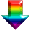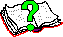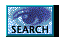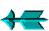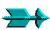\ -> Commands -> #RexxVar

 #RexxVar

This command allows you to manipulate rexx variables. You can assign values to rexx variables, save them on a stack or give them the result of simple expressions which can be calculated much faster than with a #evaluate command.

Note that you should try to pick unusual rexx variable names otherwise you might overwrite one that PPWIZARD uses. It could cause very strange behaviour.

 Syntax #1 - Set Up "X" Variable

```[WhiteSpace]#RexxVar  ["]VariableName["]  =x=   [']ItsValue[']
```

This command allows you to set up "<?xXX>" codes.

The "VariableName" parameter is the name of the 'x' variable and can contain virtually any character.

The "ItsValue" parameter is the value which the rexx variable is to contain. Unlike the "#evaluate" command the new value is not first interpreted. It is taken as is. In practice I recommend using a quote character such as "^" as its unlikely to appear in the text, this may make it easier to follow in debug mode etc. This parameter can be a variable's name (if unquoted).

 Syntax #2 - Assign to Rexx Variable

```[WhiteSpace]#RexxVar  ["]VariableName["]  =   [']ItsValue[']
```

This form of the command lets you assign text directly to a rexx variable. It is very useful in macros as you don't have to worry about quote issues while manipulating the data. For example you would find it very difficult to uppercase some text (probably passed as a macro parameter) if it contained both a single quote and a double quote and in fact how would you know which if any it contained?

The "VariableName" parameter is the full name of the rexx variable to be set.

The "ItsValue" parameter is the value which the rexx variable is to contain. Unlike the "#evaluate" command the new value is not first interpreted. It is taken as is. In practice I recommend using a quote character such as "^" as its unlikely to appear in the text, this may make it easier to follow in debug mode etc. This parameter can be a variable's name (if unquoted).

 Syntax #3 - Get value of rexx variable

```[WhiteSpace]#RexxVar  ["]VariableName["]  =value=   [']VariableName[']
```

This form of the command allows you to get the value of a variable given its name. This would normally only be used for a variable name built up out of multiple components otherwise the use of an expression of the form "<??Variable>" is much easier.

 Syntax #4 - Save/Restore Variable on Stack

```[WhiteSpace]#RexxVar  Operator ["]VariableName1["] ...   ;;New Format
[WhiteSpace]#RexxVar  ["]VariableName["] Operator        ;;Old Format
```

The "VariableName" parameter(s) are the names of all variables being pushed or popped (saved or restored).

The "Operator" parameter should be one of the following:

1. PUSH, Save rexx variable on stack.
2. POP, get last saved value from stack.

For each variable pushed there must be a corresponding pop. It allows you to use the same variable names in multiple places without danger of the value getting modified (say by a #included file).

The variable parameters of the pop command are processed in reverse order so that if you have push and pop commands with the more than one variable the variable lists do not need to be reversed by you!

 Syntax #5 - Simple Variable Manipulation

```[WhiteSpace]#RexxVar  ["]VariableName["] Operator  Value2  [SourceValue1]
```

This form of the command lets you perform some simple operations. These will be much faster than performing the same operation using #evaluate.

The "VariableName" parameter is the full name of the rexx variable. In this format the variable will be given the result of a simple expression. The value is calculated as follows:

```VariableName = SourceValue1 {Operator} Value2;
```

The "Operator" parameter should be one of the following:

2. -, Subtraction.
3. *, Multiply.
4. /, Divide.
5. //, Divide, want remainder.
6. %, Divide, want whole number.
7. || Concatenate.

The "Value2" is the value which would be added, subtracted etc from the source value.

The "SourceValue1" parameter is the the source value. By default the value comes from "VariableName".

Both Value2 & SourceValue1 can be one of the following:

• A rexx number.
• A rexx variable.
• A rexx literal.

 Example

The following is sets the rexx variable "MyVar" to the literal "ABC" (as its quoted):

```#RexxVar       'MyVar'  =  /ABC/
```

The following is sets the rexx variable "MyVar" to the contents of the variable "ABC" (as its unquoted):

```#RexxVar       'MyVar'  =  ABC
```

The following two lines both set the rexx variable "MyVar" to the value 0:

```#RexxVar       'MyVar'  =  0
#RexxVar       MyVar    =  '0'
```

The following is sets the rexx variable "MyVar" to the 3 chars (single quote, space then double quote):

```#RexxVar       'MyVar'  =  "' ""
```

Notice that in the above example double quotes are used to contain a value that contains a double quote. This is a common reason for using the "#RexxVar" command when accepting parameters in a macro.

The following gets the value of a variable made up of two parts, the first is held in the rexx variable "PageGet_KEYPREV" and te second part is the constant "_TITLE":

```#RexxVar 'Prev' =value= ^<??PageGet_KEYPREV>_TITLE^
```

The following is one line out of a larger macro, note that we have absolutely no idea what text the parameter may expand to (for example it could easily contain single quotes):

```#RexxVar       'MyVar'  =  '{\$Text}'
```

The following lines all add one to the "Counter":

```#RexxVar       Counter  +  1               ;;Counter = Counter +  1
#RexxVar       Counter  +  1 Counter       ;;Counter = Counter +  1
#RexxVar       Counter  -  "-1"            ;;Counter = Counter - -1
```

A stupid example:

```#RexxVar       Three    +  1 2             ;;Three = 2 + 1
```

The following lines append to the end of the rexx variable "Grow":

```#RexxVar       Grow  ||  ", a string"     ;;Grow = Grow || ", a string"
#RexxVar       Grow  ||  AVariable        ;;Grow = Grow || AVariable
```

The following lines could be used in a rexx header file to skip over any subroutines the header contains after all header initialization has taken place:

```;--- Initialization ---------------------------------------------------------

;--- Jump around code -------------------------------------------------------
#RexxVar   "SkipName"   =     "SkipSubroutine_<?IncludeLevel>_<?Unique>"
signal     <??SkipName>;                    ;;Jump past functions
#RexxVar   PUSH "SkipName"                  ;;Save name of label